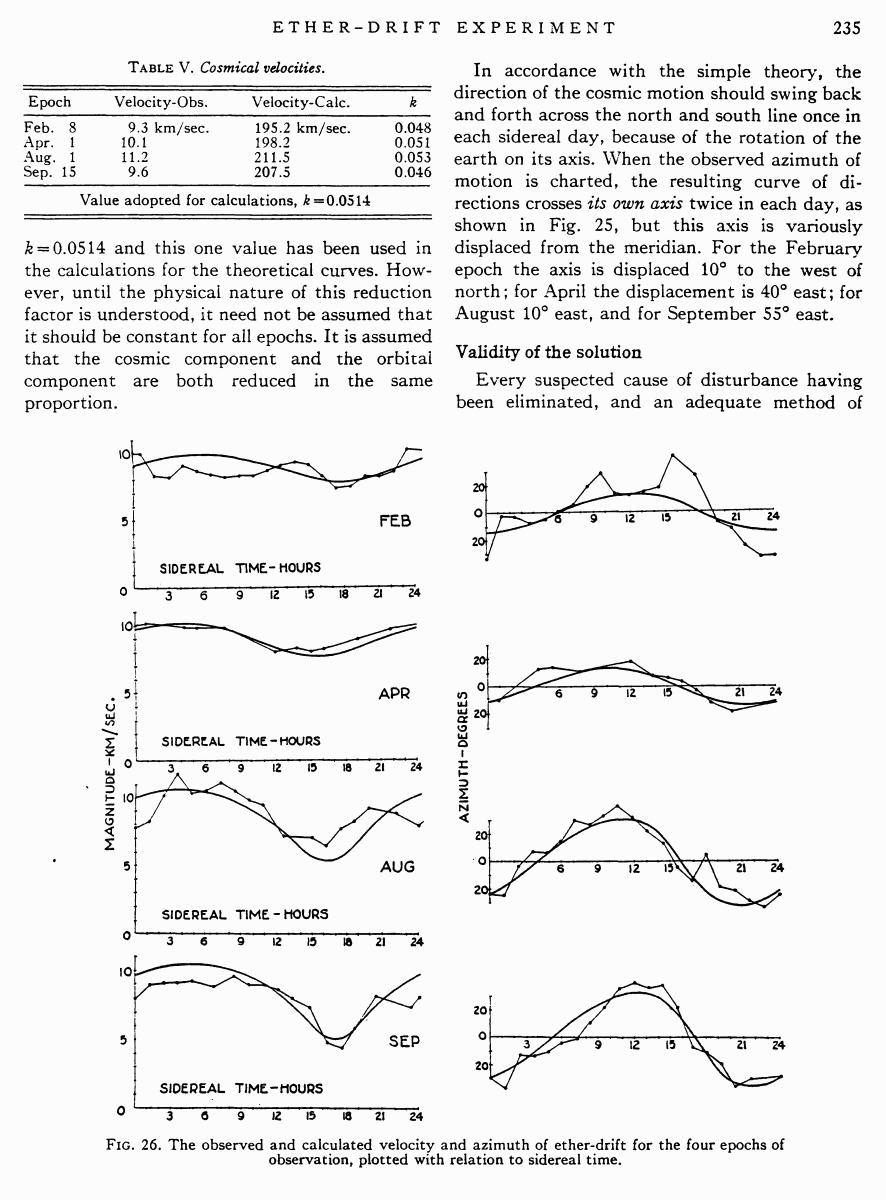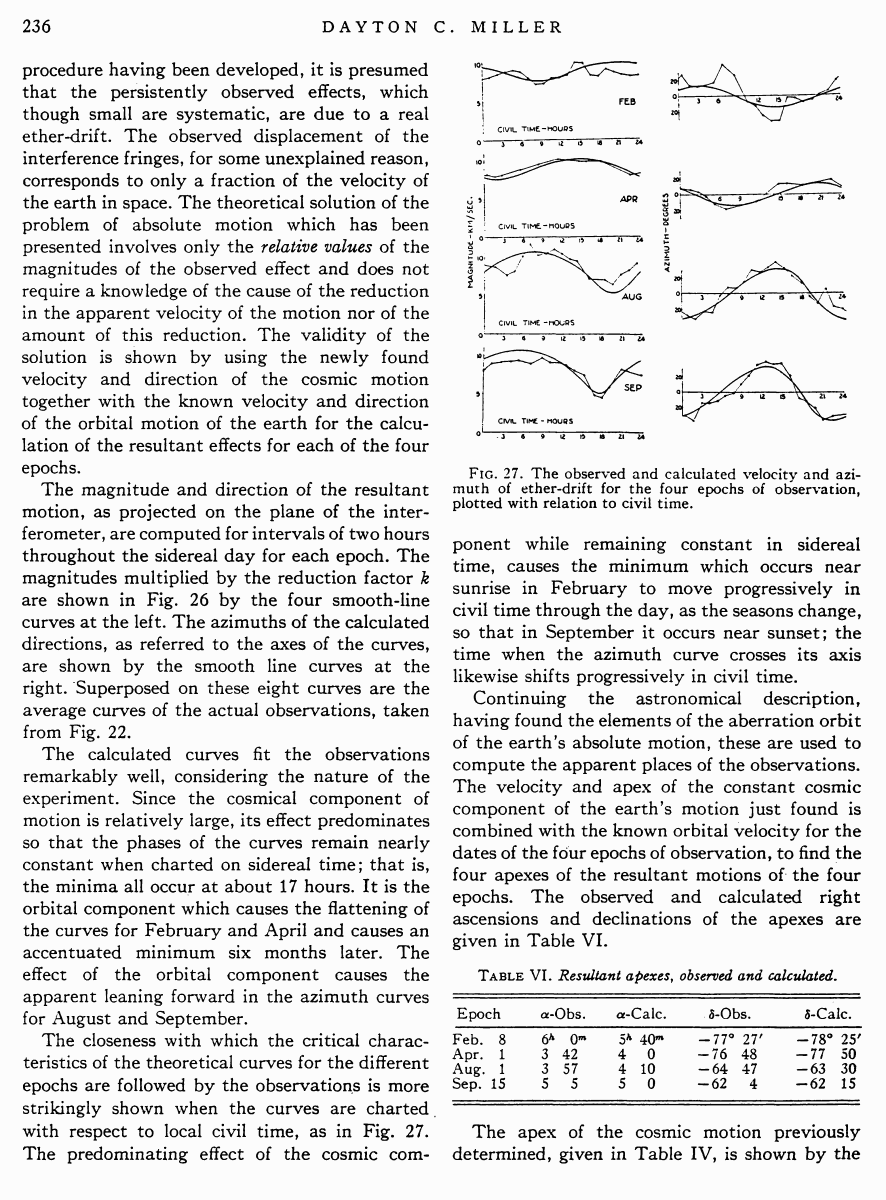# Miller D.C. The Ether-Drift Experiment and the Determination of the Absolute Motion of the Earth // Reviews of modern physics, Vol.5, July 1933

Start   PDF   <<<  Page 235   >>>203  204  205  206  207  208  209  210  211  212  213  214  215  216  217  218  219  220  221  222  223  224  225  226  227  228  229  230  231  232  233  234  235 236  237  238  239  240  241  242

Table V. Cosmical velocities.

 Epoch Velocity¢Obs. Velocity¢Calc. k Feb. 8 9.3 km/sec. 195.2 km/sec. 0.048 Apr. 1 10.1 198.2 0.051 Aug. 1 11.2 211.5 0.053 Sep. 15 9.6 207.5 0.046 Value adopted for calculations, k=0.0514

k = 0.0514 and this one value has been used in the calculations for the theoretical curves. However, until the physical nature of this reduction factor is understood, it need not be assumed that it should be constant for all epochs. It is assumed that the cosmic component and the orbital component are both reduced in the same proportion.

In accordance with the simple theory, the direction of the cosmic motion should swing back and forth across the north and south line once in each sidereal day, because of the rotation of the earth on its axis. When the observed azimuth of motion is charted, the resulting curve of directions crosses its own axis twice in each day, as shown in Fig. 25, but this axis is variously displaced from the meridian. For the February epoch the axis is displaced 10░ to the west of north; for April the displacement is 40░ east; for August 10░ east, and for September 55░ east.

Validity of the solution

Every suspected cause of disturbance having been eliminated, and an adequate method of

Fig. 26. The observed and calculated velocity and azimuth of ether-drift for the four epochs of observation, plotted with relation to sidereal time.

procedure having been developed, it is presumed that the persistently observed effects, which though small are systematic, are due to a real ether-drift. The observed displacement of the interference fringes, for some unexplained reason, corresponds to only a fraction of the velocity of the earth in space. The theoretical solution of the problem of absolute motion which has been presented involves only the relative values of the magnitudes of the observed effect and does not require a knowledge of the cause of the reduction in the apparent velocity of the motion nor of the amount of this reduction. The validity of the solution is shown by using the newly found velocity and direction of the cosmic motion together with the known velocity and direction of the orbital motion of the earth for the calculation of the resultant effects for each of the four epochs.

The magnitude and direction of the resultant motion, as projected on the plane of the interferometer, are computed for intervals of two hours throughout the sidereal day for each epoch. The magnitudes multiplied by the reduction factor k are shown in Fig. 26 by the four smooth-line curves at the left. The azimuths of the calculated directions, as referred to the axes of the curves, are shown by the smooth line curves at the right. Superposed on these eight curves are the average curves of the actual observations, taken from Fig. 22.

The calculated curves fit the observations remarkably well, considering the nature of the experiment. Since the cosmical component of motion is relatively large, its effect predominates so that the phases of the curves remain nearly constant when charted on sidereal time; that is, the minima all occur at about 17 hours. It is the orbital component which causes the flattening of the curves for February and April and causes an accentuated minimum six months later. The effect of the orbital component causes the apparent leaning forward in the azimuth curves for August and September.

The closeness with which the critical characteristics of the theoretical curves for the different epochs are followed by the observations is more strikingly shown when the curves are charted. with respect to local civil time, as in Fig. 27. The predominating effect of the cosmic com-

Fig. 27. The observed and calculated velocity and azimuth of ether-drift for the four epochs of observation, plotted with relation to civil time.

ponent while remaining constant in sidereal time, causes the minimum which occurs near sunrise in February to move progressively in civil time through the day, as the seasons change, so that in September it occurs near sunset; the time when the azimuth curve crosses its axis likewise shifts progressively in civil time.

Continuing the astronomical description, having found the elements of the aberration orbit of the earthÆs absolute motion, these are used to compute the apparent places of the observations. The velocity and apex of the constant cosmic component of the earthÆs motion just found is combined with the known orbital velocity for the dates of the four epochs of observation, to find the four apexes of the resultant motions of the four epochs. The observed and calculated right ascensions and declinations of the apexes are given in Table VI.

Table VI. Resultant apexes, observed and calculated.

 Epoch α¢Obs. α¢Calc. δ¢Obs. δ¢Calc. Feb. 8 6h 0m 5h 40m ¢77░ 27′ ¢78░ 25' Apr. 1 3 42 4 0 ¢76 48 ¢77 50 Aug. 1 3 57 4 10 ¢64 47 ¢63 30 Sep. 15 5 5 5 0 ¢62 4 ¢62 15

The apex of the cosmic motion previously determined, given in Table IV, is shown by the